﻿ Logarithmic Functions: Review and Additional Properties

#Logarithmic Functions: Review and Additional Properties

A logarithmic function is a function of the form $\,f(x) = \log_b x\,$ for $\,b \gt 0\,$ and $\,b\ne 1\,.$

The expression ‘$\,\log_b x\,$’ is read aloud as:

‘log base $\,b\,$ of $\,x\,$’

‘$\,\log_b\,$’ (read aloud as ‘log base $\,b\,$’) is the name of the function, and it acts on the input $\,x\,.$

When there's no confusion about order of operations, mathematical convention dictates that we can drop the parentheses used to ‘hold’ the input, and write ‘$\,\log_b x\,$’ (without parentheses) instead of the more cumbersome ‘$\,\log_b(x)\,$’.

If order of operations becomes ambiguous, be sure to include parentheses for clarification. Thus, for example, you should write either $\,\log_b(x + 1)\,$ or $\,(\log_b x) + 1\,,$ but never the ambiguous ‘$\,\log_b x + 1\,$’. (Notice, however, that there is no ambiguity in the expression ‘$\,1 + \log_b x\,$’.)

The number $\,b\,$ in $\,\log_b x \,$ is called the base of the logarithm.

When the base is $\,\text{e}\,,$ the function is called the natural logarithm, and can be written as $\,y = \ln x\,.$ (Note: $\text{e}\approx 2.71828\,$)

When the base is $\,10\,,$ the function is called the common logarithm, and can be written as $\,y = \log x\,.$

Logarithmic functions and their graphs were introduced in the Algebra II curriculum:

Additional higher-level information that is important for Precalculus is presented below.

## Increasing/Decreasing Properties of Logarithmic Functions

### Increasing Logarithmic Functions

When $\,b \gt 1\,,$ $\,f(x) = \log_b x\,$ is an increasing function. That is, for all positive real numbers $\,x\,$ and $\,y\,$:

$$\cssId{s23}{x \lt y \ \ \Rightarrow\ \ \log_b x \lt \log_b y}$$It is clear from the graph that the other direction is also true:

$$\cssId{s25}{\log_b x \lt \log_b y \ \ \Rightarrow\ \ x \lt y}$$

Together, we have that for all positive real numbers $\,x\,$ and $\,y\,,$ and for $\,b \gt 1\,$:

$$\begin{gather} x \lt y\cr \text{is equivalent to}\cr \log_b x \lt \log_b y \end{gather} \tag{1}$$

Notice that when the base of the logarithm is greater than one, the inequality symbols that compare the inputs ($\,x\,$ and $\,y\,$) and their corresponding outputs ($\,\log_b x\,$ and $\,\log_b y\,$) have the same direction:

$$\begin{gather} x \ {\bf\color{red}{<}}\ y\cr \text{is equivalent to}\cr \log_b x \ {\bf\color{red}{<}}\ \log_b y \end{gather} \tag{1a}$$

This equivalence can be alternatively stated as:

$$\begin{gather} x \ {\bf\color{red}{\gt}}\ y\cr \text{is equivalent to}\cr \log_b x\ {\bf\color{red}{\gt}}\ \log_b y \end{gather} \tag{1b}$$$y = \log_b x\,,$ for $\,b \gt 1\,$
An increasing logarithmic function
$x \lt y \ \iff\ \log_b x \lt \log_b y$

### Decreasing Logarithmic Functions

When $\,0 \lt b \lt 1\,,$ $\,f(x) = \log_b x\,$ is a decreasing function. That is, for all positive real numbers $\,x\,$ and $\,y\,$:

$$\cssId{s40}{x \lt y \ \ \Rightarrow\ \ \log_b x \gt \log_b y}$$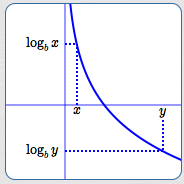It is clear from the graph that the other direction is also true:

$$\cssId{s42}{\log_b x \gt \log_b y \ \ \Rightarrow\ \ x \lt y}$$

Together, we have that for all positive real numbers $\,x\,$ and $\,y\,,$ and for $\,0 \lt b \lt 1\,$:

$$\begin{gather} x \lt y\cr \text{is equivalent to}\cr \log_b x \gt \log_b y \end{gather} \tag{2}$$

Notice that when the base of the logarithm is between zero and one, the inequality symbols that compare the inputs ($\,x\,$ and $\,y\,$) and their corresponding outputs ($\,\log_b x\,$ and $\,\log_b y\,$) have different directions:

$$\begin{gather} x \ {\bf\color{red}{<}}\ y\cr \text{is equivalent to}\cr \log_b x \ {\bf\color{red}{>}}\ \log_b y \end{gather} \tag{2a}$$

This equivalence can be alternatively stated as:

$$\begin{gather} x \ {\bf\color{red}{>}}\ y\cr \ \text{is equivalent to}\cr \log_b x \ {\bf\color{red}{<}}\ \log_b y \end{gather} \tag{2b}$$$y = \log_b x\,,$ for $\,0 \lt b \lt 1\,$
A decreasing logarithmic function
$x \lt y \ \iff\ \log_b x \gt \log_b y$

## Logarithmic Functions are One-to-One

The graphs of logarithmic functions pass both vertical and horizontal lines tests, so they are one-to-one functions. Thus, for all positive real numbers $\,x\,$ and $\,y\,$:

$$\begin{gather} x = y\cr \text{is equivalent to}\cr \log_b x = \log_b y \end{gather} \tag{3}$$

Consequently, logarithmic functions have inverses.

## Logarithmic Functions and Exponential Functions are Inverses

Logarithmic functions and exponential functions with the same bases are inverses—they ‘undo’ each other. Roughly:

• If the exponential function with base ‘$\,a\,$’ does something, then the logarithmic function with base ‘$\,a\,$’ undoes it.
• If the logarithmic function with base ‘$\,a\,$’ does something, then the exponential function with base ‘$\,a\,$’ undoes it.

These relationships are firmed up mathematically below.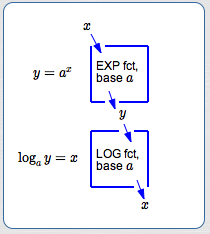Drop $\,x\,$ in the  ‘exponential function with base $\,a\,$’  box; get $\,y\,$ out the bottom. (See the top box in the diagram above.) This is expressed mathematically as:

$$\cssId{s71}{y = a^x}$$

Drop $\,y\,$ in the  ‘logarithmic function with base $\,a\,$’  box; get $\,x\,$ out the bottom. (See the bottom box in the diagram above.) This is expressed mathematically as:

$$\cssId{s76}{\log_a y = x}$$

Since the exponential and logarithmic functions with base $\,a\,$ are inverses, these two operations are equivalent:

$$\cssId{s78}{y = a^x\ \ \iff\ \ \log_a y = x}$$

If one is true, so is the other. If one is false, so is the other. They are true and false at the same time. In other words:

• If the exponential function with base $\,a\,$ takes $\,x\,$ to $\,y\,,$ then the logarithmic function with base $\,a\,$ takes $\,y\,$ back to $\,x\,.$
• If the logarithmic function with base $\,a\,$ takes $\,y\,$ to $\,x\,,$ then the exponential function with base $\,a\,$ takes $\,x\,$ back to $\,y\,.$

‘$\,y = a^x\,$’ is called the exponential form of the equation.

‘$\log_a y = x\,$’ is called the logarithmic form of the equation.The exponential and logarithmic functions with the same bases ‘undo’ each other!

When
‘EXP fct, base $\,a\,$’
takes $\,x\,$ to $\,y\,,$

then
‘LOG fct, base $\,a\,$’
takes $\,y\,$ back to $\,x\,.$

## Going from Logarithmic Form to Exponential Form

Going from logarithmic form  ‘$\,\log_a y = x\,$’  to the equivalent exponential form  ‘$\,y = a^x\,$’  is easy! Start with the base ‘$\,a\,$’, circle counterclockwise to $\,x\,,$ and then to $\,y\,$: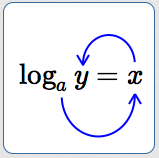This circular motion actually gives  ‘$\,a^x = y\,$’. Then, write it again from right-to-left to get ‘$\,y = a^x\,$’ !

## Going from Exponential Form to Logarithmic Form

Here's a thought process to go from exponential form  ‘$\,y = a^x\,$’   to the equivalent logarithmic form  ‘$\,\log_a y = x\,$’:

• We want a logarithmic form, so start by writing:  $\,\color{red}{\log}$
• The base of the logarithm is the same as the base of the exponential function, so add in the base:  $\,\color{red}{\log_a}$
• A logarithm is an exponent! The word ‘is’ translates as ‘$\,=\,$’.

The only exponent in  ‘$\,y = a^x\,$’  is $\,x\,.$

Leaving a space for the input, add in ‘$\,= x\,$’: $$\color{red}{\log_a\ \ \ = x}$$

• There's only one variable left in  ‘$\,y = a^x\,$’ , and only one place to put it!

Finish by putting in the ‘$\,y\,$’ : $$\color{red}{\log_a y= x}$$

## Domain/Range Considerations for Logarithmic and Exponential Functions

Let $\,a \gt 0\,,$ $\,a \ne 1\,.$ These are the allowable bases for logarithmic and exponential functions.

For exponential functions, $\,y = a^x\,$:

• the domain is the set of all real numbers
• the range is the set of positive real numbers

For logarithmic functions, $\,y = \log_a x\,$:

• the domain is the set of positive real numbers
• the range is the set of all real numbers

Observe that:

• the domain of $\,y = a^x\,$ is the range of $\,y = \log_a x\,$
• the range of $\,y = a^x\,$ is the domain of $\,y = \log_a x\,$

The input/output sets for inverses are switched! The input set for one is the output set for the other. The output set for one is the input set for the other.

## Two Important Views of Logarithms

Now, you should be comfortable with both important views of logarithms:

•   What is $\,\log_a x\,$?

It is the power (the exponent) that ‘$\,a\,$’ must be raised to, in order to get $\,x\,.$

• Logarithms ‘Undo’ Exponents:  If the exponential function with base $\,a\,$ takes input $\,x\,$ to output $\,y\,,$ then what function takes $\,y\,$ back to $\,x\,$?

Answer: the logarithmic function with base $\,a\,$

## Solving Inequalities Involving Logarithmic Functions

Equivalences (1), (2), and (3) can be used to solve certain types of mathematical sentences involving logarithms, as illustrated in the following examples.

IMPORTANT: Whenever you have an expression of the form ‘$\,\log_a (\text{stuff})\,$’, the ‘stuff ’ must be positive!

### Example 1

SOLVE:

$$\cssId{sb47}{\log_2(3x-1) \lt \log_2(5x)}$$

This is an inequality of the form

$$\cssId{sb49}{\log_b(\text{stuff1}) \lt \log_b(\text{stuff2})}$$

where the base of the logarithm, $\,b\,,$ is $\,2\,.$

Notice that the logarithmic functions on both sides use the same base.

In this example, the base is greater than one, so we'll use equivalence $(1)$:

$$\cssId{sb53}{x \lt y \ \ \iff\ \ \log_b x \lt \log_b y}$$

SOLUTION:

$\log_2(3x-1) \ \lt \ \log_2(5x)$
Original inequality
$3x-1 \ \lt \ 5x$
Use (1); inequality symbol doesn't change
$-2x \lt 1$
$x \gt -\frac 12$
Divide by a negative #; reverse inequality

Since logarithms only act on positive numbers, we must also have:

$$\begin{gather} 3x - 1 \gt 0 \quad \text{and} \quad 5x \gt 0\cr x \gt \frac 13 \quad \text{and} \quad x \gt 0 \end{gather}$$

Putting everything together:

$$\begin{gather} x \gt -\frac 12 \ \ \text{and} \ \ x \gt \frac 13 \ \ \text{and} \ \ x \gt 0\cr \text{is equivalent to}\cr x \gt \frac 13 \end{gather}$$

The solution set of this inequality is:

$$\cssId{sb61}{\underbrace{\{x\ |\ x > \frac 13\}}_{\text{set-builder notation}} = \underbrace{(\frac 13,\infty)}_{\text{interval notation}}}$$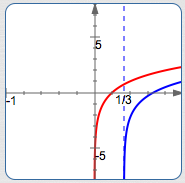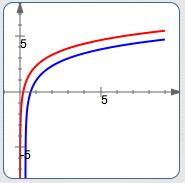Blue curve:  $y = \log_2 (3x - 1)$

Red curve:  $y = \log_2 (5x)$

The blue curve is only defined for $\,x \gt \frac 13\,.$

The blue curve lies below the red curve everywhere that it is defined.

Head up to wolframalpha.com and type in:

log_2 (3x -1) < log_2 (5x)

Voila!

### Example 2

SOLVE:

$$\cssId{sb71}{\log_{0.3}(7) - \log_{0.3}(1 - 6x) \gt 0}$$

This is an inequality that can be easily transformed to

$$\cssId{sb73}{\log_b(\text{stuff1}) > \log_b(\text{stuff2})}$$

where the base of the logarithm, $\,b\,,$ is $\,0.3\,.$ Notice that the logarithmic functions use the same base.

In this example, the base is between zero and one, so we'll use equivalence $(2)$:

$$\cssId{sb77}{x \lt y \ \ \iff\ \ \log_b x \gt \log_b y}$$

Compare the solution below to what you get at wolframalpha.com:

log_(0.3) (7) - log_(0.3) (1 - 6x) > 0

SOLUTION:

$$\begin{gather} \log_{0.3}(7) - \log_{0.3}(1 - 6x) \gt 0\cr\cr \log_{0.3}(7) \gt \log_{0.3}(1 - 6x)\cr\cr 7 \lt 1 - 6x \ \ \text{and}\ \ 1 - 6x \gt 0\cr\cr 6x \lt -6 \ \ \text{and}\ \ -6x \gt -1\cr\cr x \lt -1 \ \ \ \text{and}\ \ \ x \lt \frac 16\cr\cr x \lt -1 \end{gather}$$

Here are the reasons for each step:

• Original inequality
• Equivalence $(2)$—inequality symbol changes direction; inputs to logs must be positive
• Multiplication property for inequalities
• How can a number be both less than $\,-1\,$ and less than $\,\frac 16\,$ at the same time?

Answer: By being less than $\,-1\,$!

Notice in the third step that the inequality symbol changed direction, since equivalence $(2)$ is being used.

### Example 3

SOLVE:

$$\cssId{sb94}{\ln(2x + 1) = \ln(7x - 3)}$$

Here's a screenshot from wolframalpha.com: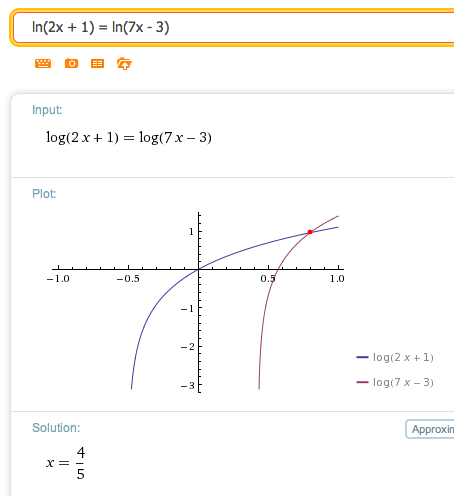Writing a list of equivalent mathematical sentences:

$$\begin{gather} \ln(2x + 1) = \ln(7x - 3)\cr\cr 2x + 1 = 7x - 3\cr \text{and}\ \ 2x + 1 \gt 0 \ \ \text{and}\ \ 7x-3 \gt 0\cr\cr -5x = -4\cr \text{and}\ \ 2x \gt -1 \ \ \text{and}\ \ 7x \gt 3\cr\cr x = \frac 45\cr \text{and}\ \ \ x \gt -\frac 12 \ \ \ \text{and}\ \ \ x \gt \frac{3}{7}\cr\cr x = \frac 45 \end{gather}$$